# HP 50g Calculator - The Basics of Plotting Functions

## Plotting on the HP 50g

The HP 50g calculator provides a host of plots to allow the user to visualize data or relationships between them. The white shifted-functions of the top row of keys on the HP 50g allow access to many of the input forms where plotting specifications may be entered.

## 2D/3D (PLOT SETUP) form

This example is performed in RPN mode. To set RPN mode:

1. Press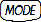.
2. With the field to the right of ‘Operating Mode’ highlighted, press the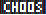menu key. Use the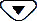key to highlight ‘RPN’ in the Choose Box.
3. Press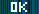.
4. Pressagain to return to the ‘HOME’ screen.
The 2D/3D (PLOT SETUP) form is accessed by pressing left shift,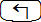, and simultaneously pressing and holding the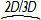(F4) key. When pressed, a form titled ‘PLOT SETUP’ is displayed with a number of choices related to plotting as shown in following figure.

Figure 1: PLOT SETUP screen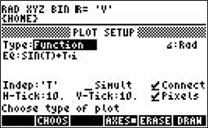NOTE: To access the 'PLOT SETUP' form using an emulator, right-click left shift key, (), followed by a left-click of.
The first option allows selecting the plot type from a number of options. The selections for plot type are displayed by pressing themenu key. The plot types include:
 Functions Scatter charts Polar plots Slopefield charts Parametric plots Fast 3D charts Differential equation plots Wireframe plots Conic plots Ps-contour plots Truth plots Y-slice plots Histograms Gridmap plots Bar charts Pr-surface plots
After pressing themenu key, a choose box appears as shown below in Figure 2. Use the cursor keys to highlight the option and press. For this example, use the ‘Function’ option.

Figure 2: CHOOSE box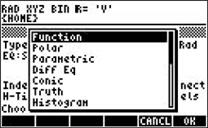The ‘PLOT SETUP’ form also allows the user to specify the equation being plotted. Place the cursor on the ‘EQ:’ field using the cursor keys and press the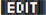menu label to invoke the EquationWriter used for constructing the equation to be plotted. See Figure 3.

Figure 3: EquationWriter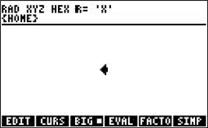With the EquationWriter screen displayed, key in the desired equation using the keypad and the right-arrow cursor key (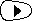) to move from one part of the equation to another. Press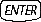to input the equation.

For example, to enter y = x2-4, with ‘Function’ highlighted in the ‘PLOT SETUP’ screen, press:

1.and themenu key to invoke the EquationWriter.
2. With the EquationWriter open, as shown in Figure 3, press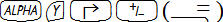.
3. Press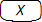(the X key)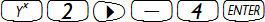.

This returns to the ‘PLOT SETUP’ screen with the equation in the 'EQ:' field. The form also allows to change the angle measure and the independent variable to be specified.

NOTE: The default is often 'X,' but for parametric plots, this will be changed to ‘t’.

For this example, be sure the independent variable (Indep:) is ‘X’. In addition, several check boxes indicate whether the plotted points should be automatically connected together by the calculator and the settings for the horizontal and vertical tick marks used for the graph. The form also allows for the plotting of more than one function at a time. For this example, leave all these settings in their defaults.

## The WIN form

The WIN form allows for the ‘PLOT WINDOW’ specifications to be entered and changed. The lower and upper horizontal and vertical values displayed on the graph can be changed. The lower and upper value for the independent variable can also be specified on this form. Use the arrow keys to move around the form.

To open the WIN form, pressfollowed by the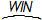(F2) key.

Figure 4: PLOT WINDOW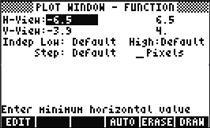In the fields of the ‘PLOT WINDOW’ screen, enter the desired upper and lower values for the plot followed by. For this example, set the x values from -3 to 3 (in the fields 'Indep Low:' and 'High:') and the y values -6 to 6 (in the fields 'H-View:' and 'V-View:').

With the ‘PLOT WINDOW’ displayed, and the cursor to the right of 'H-View:', press:

1.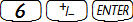2. Press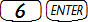3. Press4. Press5. Press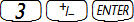6. Press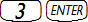.

Figure 5: PLOT WINDOW with values entered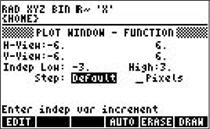Once these parameters have been updated, press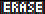to discard the results of a previous plot, and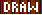to begin the plot. The plot should appear in the display as shown in the following figure. Note the new menu options.

Figure 6: PLOT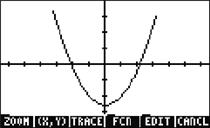## What Can I Do with the Plot?

1. Use the arrow keys to move the cursor around the plot. Press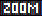to open a zoom menu and zoom in and out, select a region using a box, etc. Press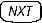to see more options. Press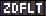(zoom default) to return to the default zoom size, but note that these settings differ from those entered for the previous example. To leave the Zoom menu, pressuntil the menu option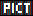is displayed. Press it to return to the main plot screen and menu. To re-enter the values for the plot window used in this example, press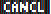to exit the graph screen and open the 'PLOT WINDOW'. Once the values are entered, pressto discard the results of a previous plot, andto plot.
2. Press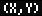to move the cursor along the graph and read the x-y coordinates with the cursor keys. Pressto return to the main plot menu.
3. Once back to the main plot screen, press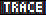. The small white box to the left of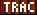indicates the tracer is active. With the Tracer active, the cursor traces along the function by pressing the left and right arrow keys.
4. Press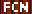for functions for finding roots, areas, intersections, etc. For example, press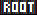and the closest root will be calculated. Press thekey so the menu appears again, thenagain to see more options. Pressto return to the main plot window.
5. Pressto access functions for marking points, drawing, and creating lines in the graph. Pressagain to see more options, andto return to the main plot window.
6. Pressto exit the graph screen.
7. From here, pressfollowed by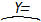(the F1) key to return to the 'PLOT FUNCTION' screen. Select the top function(s) (the derivatives) and pressto delete any entries, if any, from the previous steps so that only the original equation remains, y=x2-4.
8. Now pressfollowed by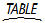(F6) key to enter the table. Here view the table of the 'X' and function values. Select an item in the 'X' column and type 1.215.
9. Pressand note the table jumps to that value and displays again.
10. Pressfollowed by(F2) key to return to the 'PLOT WINDOW' screen, orfollowed by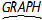(F3) key to return to the graph. Press the CANCEL key (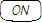) on the keyboard to return to the 'HOME' screen.